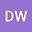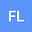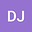Modelling hillslope sediment transport rate on grassland
•••• +3
• Dongdong Wang,
• Fen Liu,
• Dawei Jing,
• Yan Tang,
• Ningning Song,
• Zaijian Yuan
Dongdong Wang
College of Resources, Environment and Planning
Author ProfileFen Liu
College of Resources, Environment and Planning
Author ProfileDawei Jing
College of Resources, Environment and Planning
Author ProfileYan Tang
Dezhou University
Author ProfileNingning Song
Dezhou University
Author ProfileZaijian Yuan
Dezhou University
Author Profile## Abstract

Few data are available for estimating the sediment transport rate on a steep slope of grass with different covers. In this study, the artificial simulated rainfall test is used to investigate how rainfall intensity, slope and cover affect the sediment transport rate. Simultaneously, the study establishes a model for the sediment transport rate using shear stress, stream power, unit stream power and unit energy on steep grassland slopes. Results show that the sediment transport rate decreases as the vegetation cover increases, as described by linear or logarithmic equations under different rainfall intensities or slopes. The sediment transport rate increases as an exponential function equation with rainfall intensity, slope and cover with a Nash–Sutcliffe model efficiency (NSE) value of 0.864. The effects of slope steepness are stronger than the effects of rainfall intensity and cover. Regression analyses show that the sediment transport rate can be predicted from the power function equations of shear stress, stream power and unit energy. In addition, the sediment transport rate can be fit to unit stream power with linear equation (NSE = 0.840). However, shear stress, stream power and unit energy perform poorly (NSE = 0.394, NSE = 0.498 and NSE = 0.330, respectively). Further analysis shows that the sediment transport rate is best modelled by a power function equation that includes three factors, i.e. rainfall intensity, vegetation cover and slope. Moreover, unit stream power results in the best model for the sediment transport rate among the different hydrodynamic parameters. The soil erodibility parameter and critical unit stream power of this experiment are 113.59 and 0.216 m·s-1, respectively, which are six times more than those in the bare slope. The measurements and calculations of the sediment transport rate, the calculations of the surface roughness and characteristic considerations of the vegetation for sheet flow should be explored in future research, which are important in improving experimental accuracy and sediment transport rate modelling